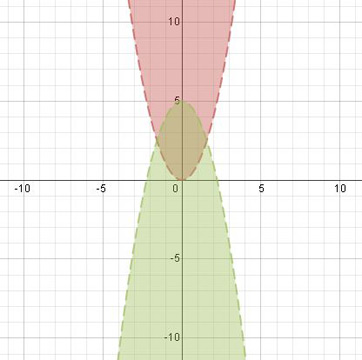# Graphing a System of Quadratic Inequalities: Examples & Process

Instructions:

Choose an answer and hit 'next'. You will receive your score and answers at the end.

question 1 of 3

### What part is shaded for the graph of y < x^2 - 1?

Create Your Account To Take This Quiz

As a member, you'll also get unlimited access to over 75,000 lessons in math, English, science, history, and more. Plus, get practice tests, quizzes, and personalized coaching to help you succeed.

Try it risk-free for 30 days. Cancel anytime.

### 2. Which part of this graph is the solution region?Create your account to access this entire worksheet
A Premium account gives you access to all lesson, practice exams, quizzes & worksheets
Quizzes, practice exams & worksheets
Certificate of Completion
Create an account to get started

This quiz and corresponding worksheet will help you gauge your knowledge of how to graph a system of quadratic inequalities. You will be taking a look at how to find the common solution areas to a function that may not be exactly equal. Topics you'll need to know to pass the quiz include understanding which part of a graph would be shaded for y < x^2 - 1 as well as knowing what kind of inequality is used when the line is solid and the shading is above the line.

## Quiz & Worksheet Goals

Use this printable worksheet and quiz to review:

• Shading for y < x^2 - 1
• Inequality used for a solid line and the shading above it
• Solution region for a given problem
• Symbol of inequality used
• How a system of functions is symbolized

## Skills Practiced

This worksheet and quiz will let you practice the following skills:

• Interpreting information - verify you can read information regarding how a system of functions is symbolized and interpret it correctly
• Problem solving - use acquired knowledge to solve various quadratic inequality graph problems
• Reading comprehension - ensure that you draw the most important information from the related graphing a quadratic inequality lesson

Make sure to also read the accompanying lesson called Graphing a System of Quadratic Inequalities: Examples & Process. This lesson will help you:

• Define what a quadratic inequality is
• Identify how the inequality relates to a system of quadratic inequalities
• Understand how to mathematically notate the system
• Describe what happens if the inequality is less than or less than or equal to
• Appreciate what the overlapping shading on the graph represents
Final ExamMath 105: Precalculus Algebra
Status: Not Started
Chapter Exam